#0

## [R語言]資料分析讀書心得05---使用dplyr做資料變換(filter,arrange,缺失值)

``````library(nycflights13)
library(tidyverse)
``````

``````glimpse(flights)
``````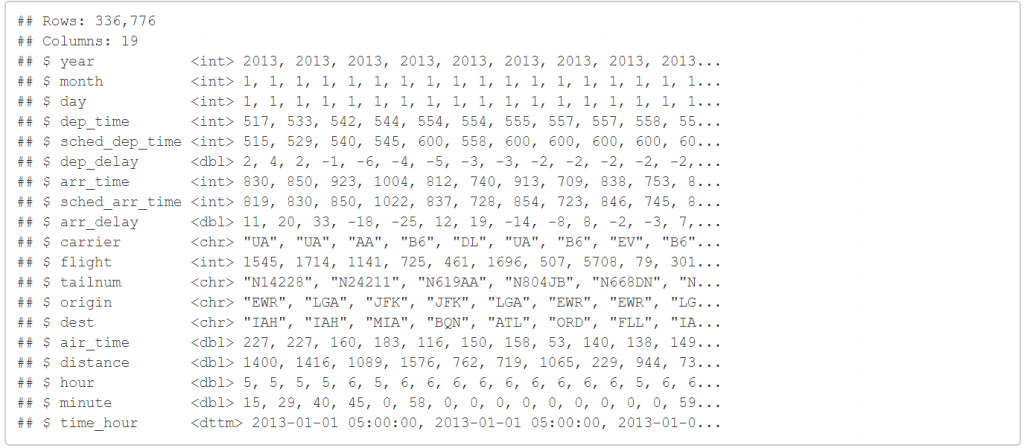1. int:代表整數
2. dbl:代表雙精度浮點數
3. chr:代表字串
4. dttm:代表日期與時間
5. lgl:代表邏輯向量
6. fctr:代表因子
7. date:代表日期

flights 是航班資料，如果今日我只想要看 1/1 的資料，我們可以試試看以下語法。

``````filter(flights,month==1,day==1)
``````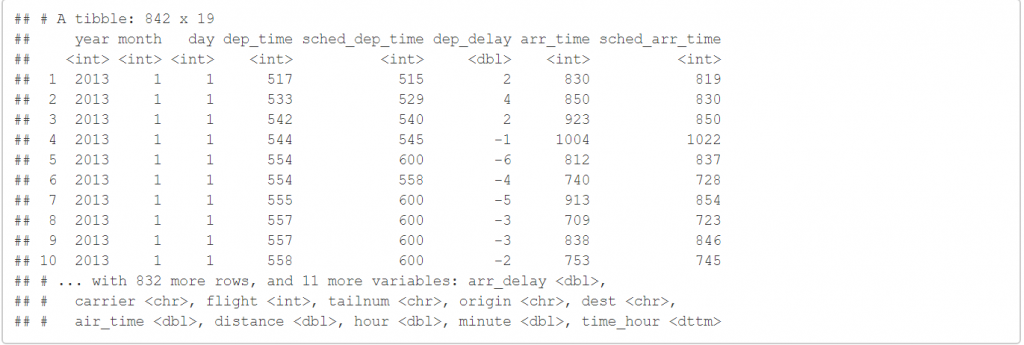``````filter(flights,month==11|month==12)
``````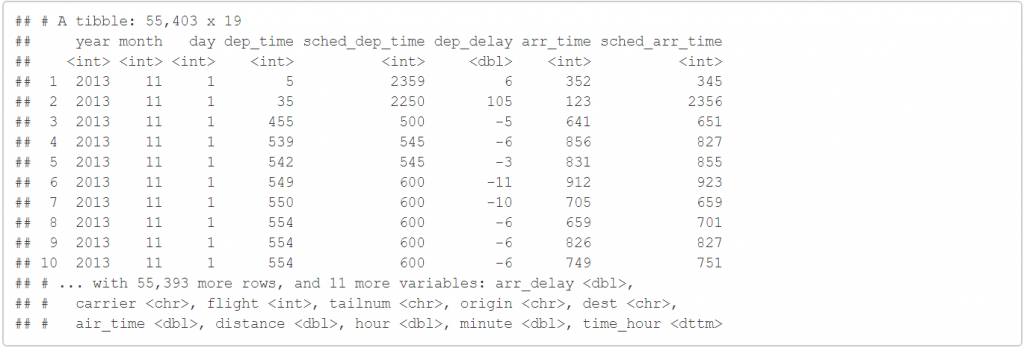``````arrange(flights,year,month,day)
``````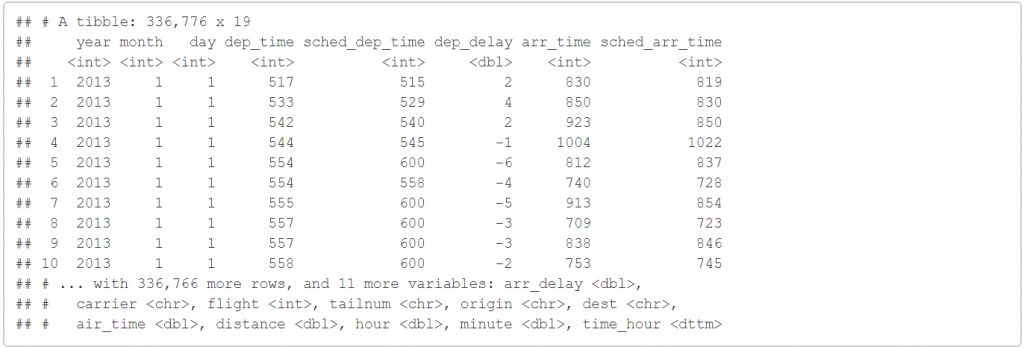``````arrange(flights,desc(arr_delay))
``````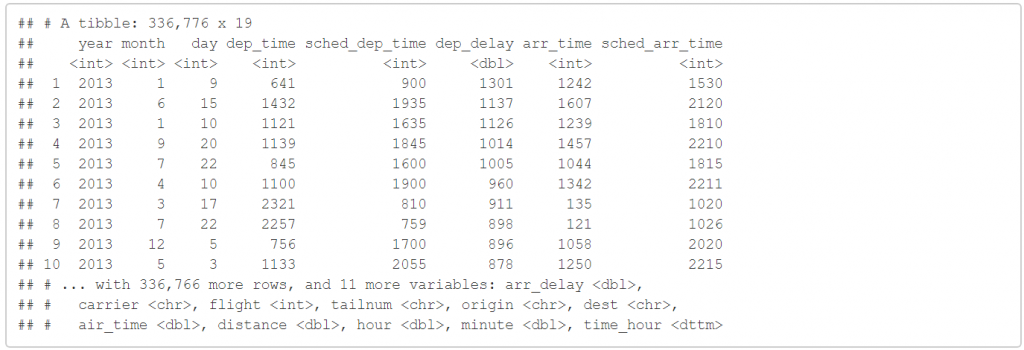``````NA==NA
``````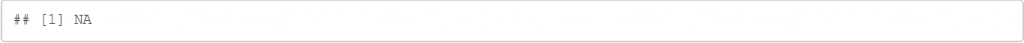``````class(pi)
``````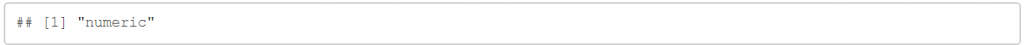``````class(TRUE)
``````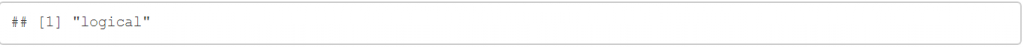NA 到底是何種資料呢?

``````class(NA)
````````````NA+5
````````````NA>5
````````````class(NA+5)
````````````class(NA>5)
````````````is.na(NA)
``````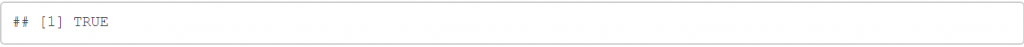``````is.na(NA>6)
``````